# Fantastic Maths

Year One have been working really hard in Maths this week. This week we have been adding and subtracting. We have been adding and subtracting using a number-line to help us. Some of us have also been subtracting by crossing off representations to find the answer.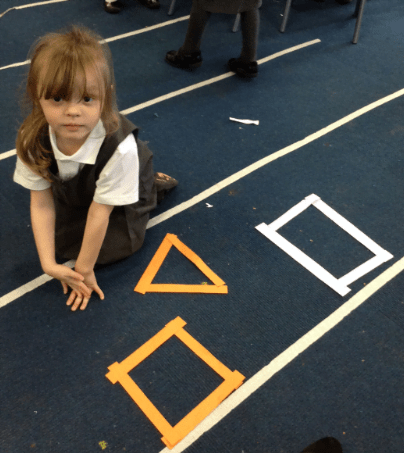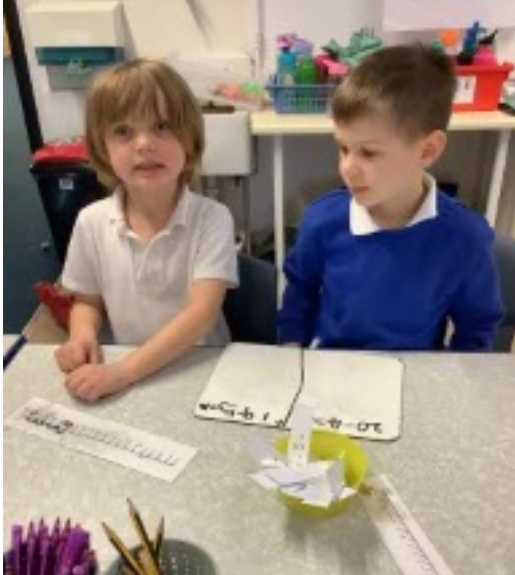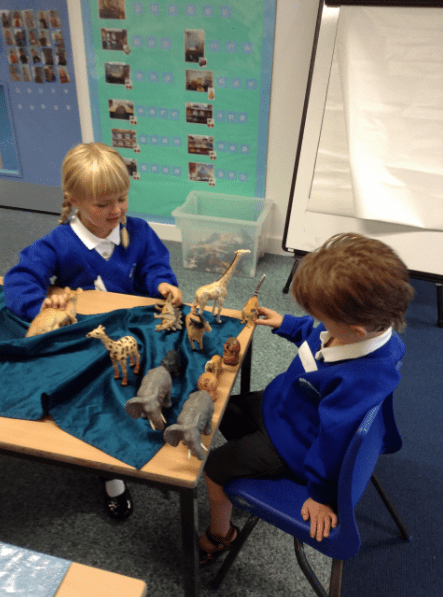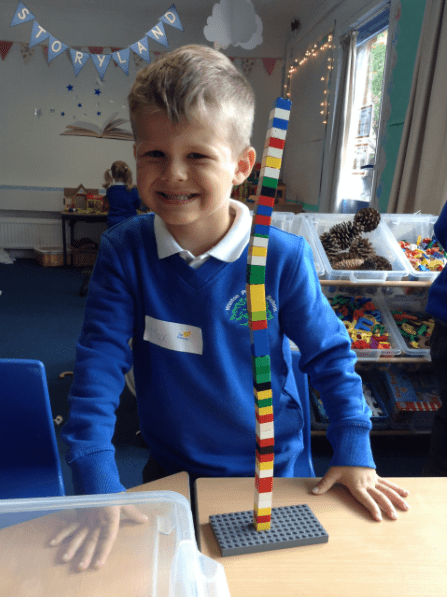Following this, we moved on to working out addition calculations which cross ten. We did this by using a tens frame and counters and combining the groups to make ten first. We then were able to work out the answer more efficiently. After using concrete resources to help us we moved on to drawing this pictorially.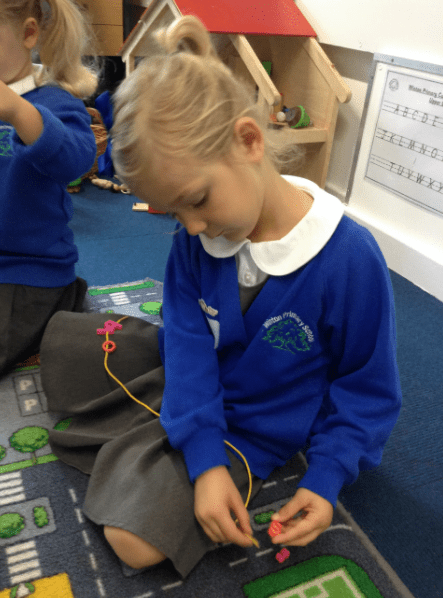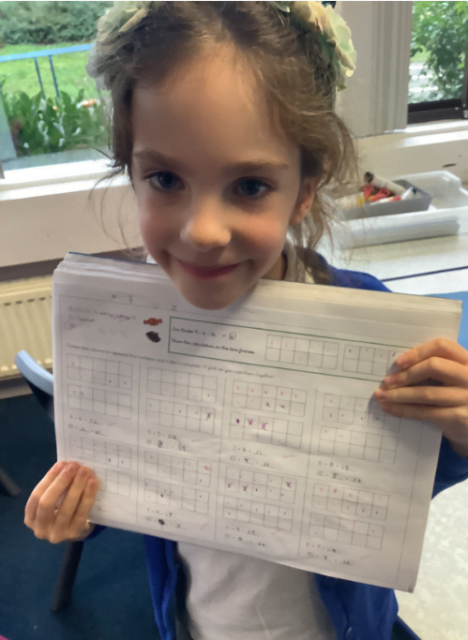Finally, we moved on to working out subtraction calculations which cross ten by using a number line. This time we used our number bond knowledge to ‘jump’ back to ten first and then continued to count back to find the answer. This was quite tricky! But the teachers have been really pleased with how well the children have persevered. To finish off the week we have been writing addition and subtraction calculations for pictorial representations.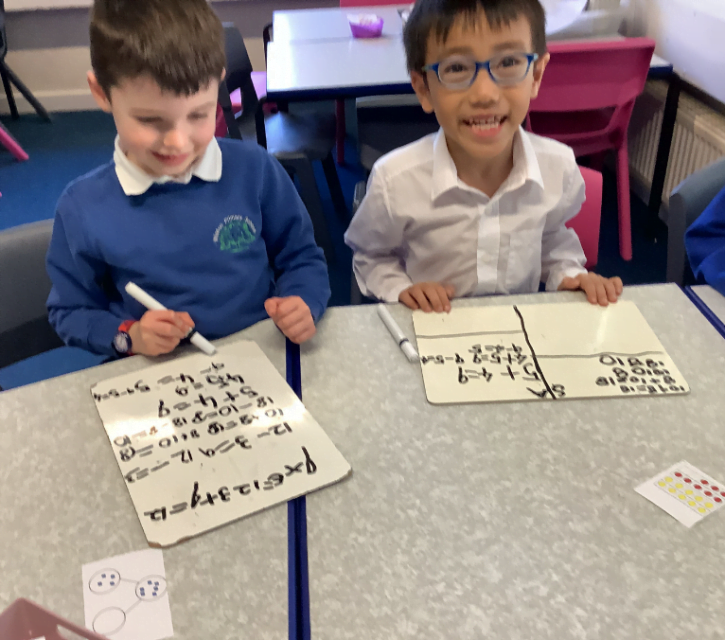Well done Year One! A great week in Maths and some fantastic work to show for it.